## 【内排序 -- 八大排序】

news/2023/6/7 0:45:50

### 目录：

• 前言
• 算法实现
• （一）插入排序
• 1.直接插入排序
• 2.希尔排序（缩小增量排序）
• （二）选择排序
• 1.选择排序
• 2.堆排序
• （三）交换排序
• 冒泡排序
• 快速排序1（hoare版）
• 快速排序2（挖坑版）
• 快速排序3（前后指针版）
• 优化
• ①三数取中
• ②小区间优化
• 快速排序4（非递归版）
• （四）归并排序
• 递归版
• 非递归版
• （五）计数排序
• 三、性能分析
• 总结

# 前言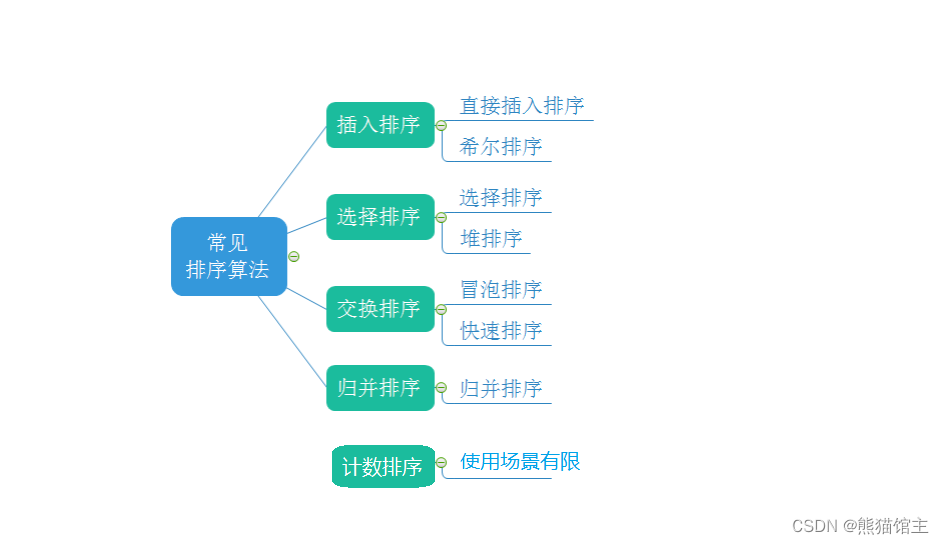# 算法实现

## （一）插入排序

### 1.直接插入排序

1. 将待排序数据（aim）插入到有序序列中；
2. 大于aim的数据都往后移；
3. 将aim插入到小于等于它的数据之后。``````// 插入排序  时间复杂度：O(n^2)   --  稳定排序
void InsertSort(int* a, int n)
{for (int i = 1; i < n; i++)  //   需要向前插入的数据下标{int aim = a[i];   //  保存目标数据int j = 0;for (j = i - 1; j >= 0; j--){if (a[j] > aim)    //  如果a[j] 大于 aim， 就将a[j] 向后移动一位a[j + 1] = a[j];else               //   否则就是找到了目标位置，结束循环break;}//   情况0：第一次就不需要移动，此时aim仍然在原位置，也就是 j+1a[j + 1] = aim;       //   情况1：找到了合适位置：在下标j之后的位置，也就是 j+1//    情况2：一直循环到 j == -1 的位置，此时应该将aim放到 j = 0 的位置上，也就是 j+1}
}
``````

5. 元素集合越接近有序，直接插入算法时间效率越高
6. 时间复杂度：O(N^2)
7. 空间复杂度：O(1)
8. 稳定性：稳定

### 2.希尔排序（缩小增量排序）

1. 选定一个整数gap = n / 2，然后将数据分成gap组；
2. 所以间隔为gap的数据分在一组，每组数据进行插入排序（预排序）；
3. 令gap /= 2，重复上述操作；
4. 直到gap=1，此时所有数据分到一组，进行直接插入排序。 （上面所说肯定会让大家有很多疑问，这里说明一点：gap为数据之间的跨度，在直接插入排序中gap就是1，每次都是和前面间隔为1（gap）的数据进行比较，
而希尔排序会先扩大gap的值，这样就可以将数据更快地移动到合适的位置）``````// 希尔排序    --   预排序   不稳定排序
void ShellSort(int* a, int n)
{//  确定跨度int gap = n / 2;   //  也可写作：gap = n/3 + 1;  保证最后gap能够变为1while (gap >= 1){int k = 0;while (k < gap){for (int i = gap; i < n; i += gap)  //  插入排序{int cnt = a[i];int j = 0;for (j = i - gap; j >= 0; j -= gap){if (a[j] > cnt)a[j + gap] = a[j];elsebreak;}a[j + gap] = cnt;}k++;}gap /= 2;}}//  由于四层循环不容易控制，这里我们进行了简化，如果大家有兴趣可以画一画图来加深理解
void ShellSort(int* a, int n)
{//  确定跨度int gap = n / 2;   //  也可写作：gap = n/3 + 1;  保证最后gap能够变为1while (gap >= 1){// int k = 0;// while (k < gap)   //   可合并化简，省去一层循环（表面上）// {// for (int i = gap; i < n; i += gap)for (int i = gap; i < n; i++){int cnt = a[i];int j = 0;for (j = i - gap; j >= 0; j -= gap){if (a[j] > cnt)a[j + gap] = a[j];elsebreak;}a[j + gap] = cnt;}//	k++;//	}gap /= 2;}}
``````

gap=n/2 可以， gap=n/4+1 可以，gap=gap-1等等亦可以，也就是说只要能够保证最后gap=1就都是可行的，

1. 时间复杂度：O(N^1.3)
2. 空间复杂度：O(1)
3. 稳定性：不稳定

## （二）选择排序

### 1.选择排序

1. 遍历数据，找到最大数，并记录最大数据的下标；
2. 将最大值换到最后；
3. i++，重复上述操作``````// 选择排序 - 1     每次选择一个最大数放到最后
void SelectSort(int* a, int n)
{for (int i = 0; i < n - 1; i++){int sub = 0;  //  记录最大值下标for (int j = 1; j < n - i; j++){if (a[j] > a[sub])sub = j;}Swap(a + sub, a + n - 1 - i);}
}// 选择排序 - 2  优化 ：一次找两个值
void SelectSort(int* a, int n)
{for (int i = 0; i < n / 2; i++)      //   注意 i ，j 的取值范围的改变{int lsub = i;   //   最小值下标int rsub = i;   //   最大值下标for (int j = i + 1; j < n - i; j++){if (a[j] < a[lsub])lsub = j;if (a[j] > a[rsub])rsub = j;}if (rsub == i)  //  最大值位于第一个位置，那么下面在将最小值换到第一个位置的时候会将最大值换到lsub的位置，rsub = lsub;  //  因此需要更新rsub下标Swap(a + i, a + lsub);                     //  交换函数请自行实现Swap(a + n - 1 - i, a + rsub);}
}
``````

1. 无论数据是否有序，选择排序的时间复杂度都为O(N^2)，因此在现实生活中很少使用。
2. 时间复杂度：O(N^2)
3. 空间复杂度：O(1)
4. 稳定性：不稳定

### 2.堆排序

1. 利用完全二叉树的特性，每次从最后一个父亲节点开始与孩子节点进行比较，将最大值换到根节点；（向下调整）
2. 将最大值换到最后；
3. 经过了上一步的交换，根节点可能已经不是最大值，就需要将根节点从新与孩子节点进行比较，将最大值换到根节点；
4. 重复2，3两个步骤。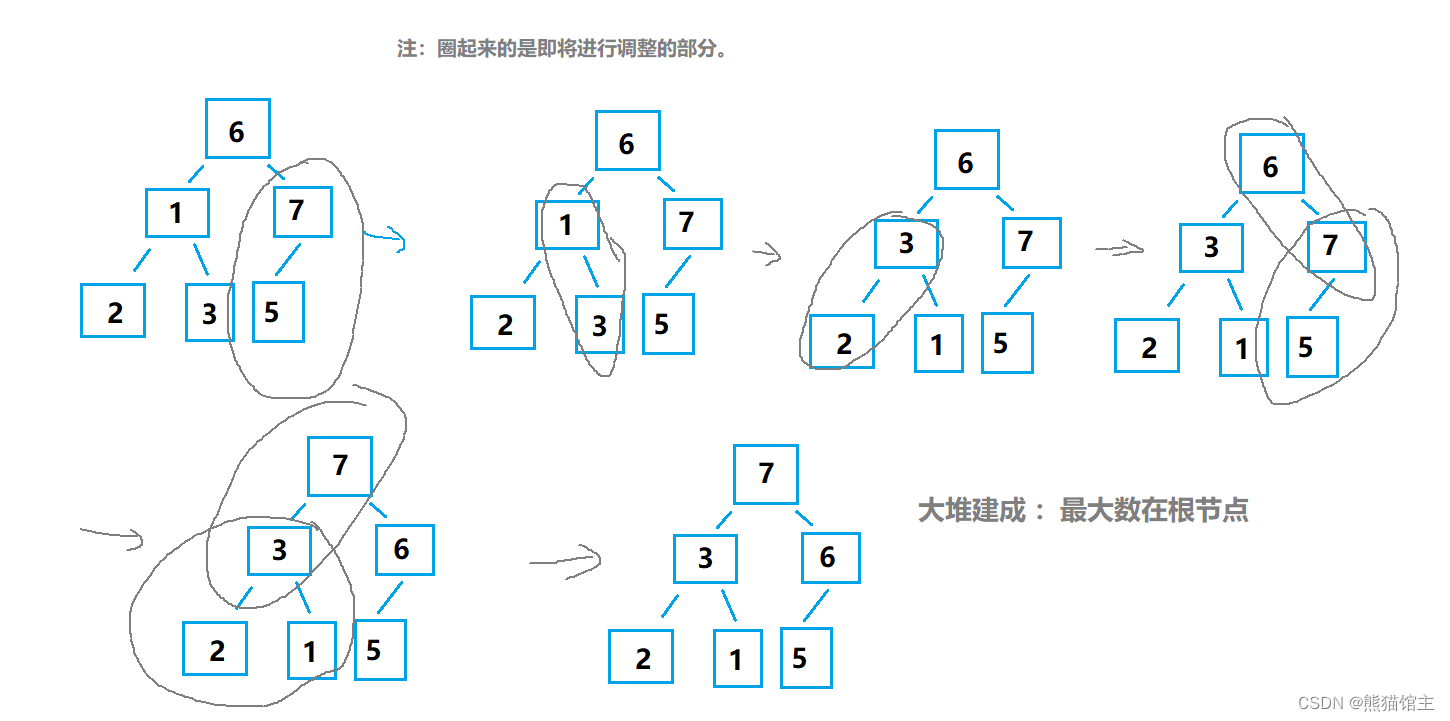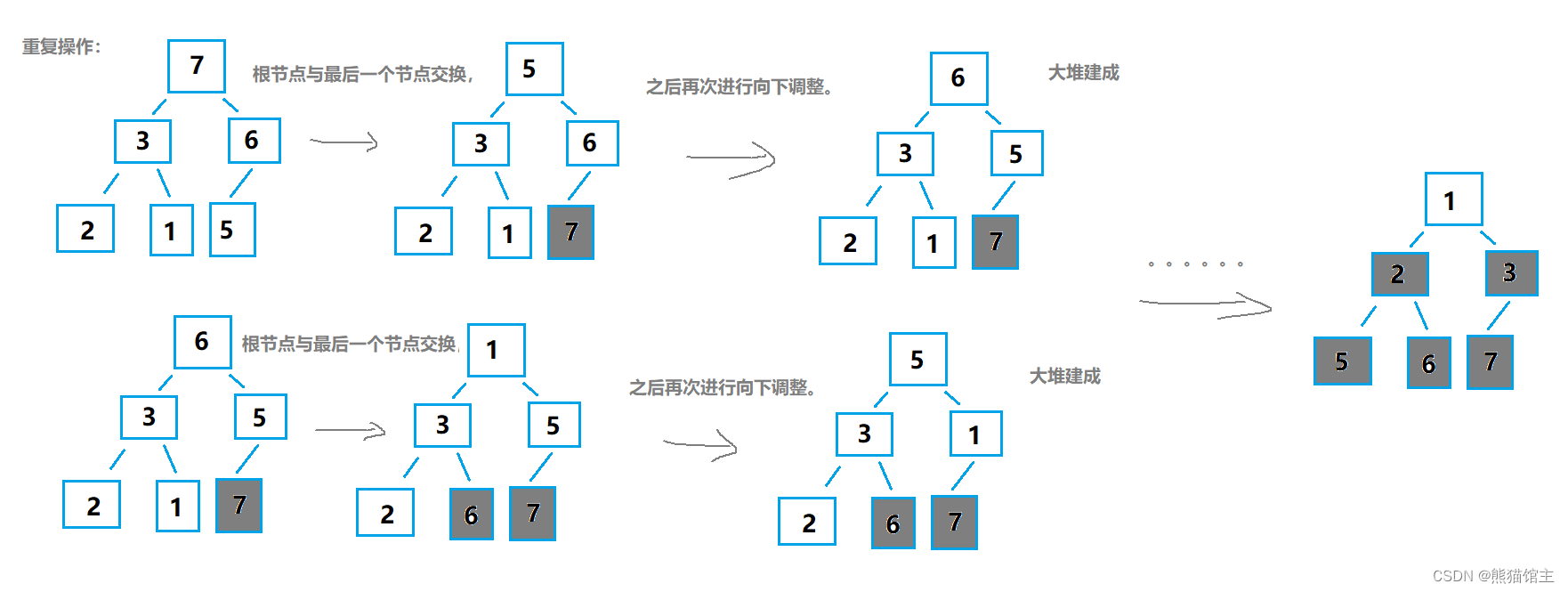``````typedef int HPDaataTyep;
typedef struct Heap
{HPDaataTyep* a;int size;int capacity;
}Heap;// 堆排序   --升序排序建大堆
void AdjustDown(int* a, int n, int root)
{int parent = root;int child = root * 2;while (child < n){if (child + 1 < n && a[child + 1] > a[child])  // 右孩子存在且更大child++;if (a[child] > a[parent])  //  孩子大于双亲--交换{Swap(a + child, a + parent);parent = child;child = parent * 2;}else{break;}}
}void HeapSort(int* a, int n)
{Heap hp;hp.a = a;hp.size = hp.capacity = n;for (int i = n / 2; i >= 0; i--)  // 从第一个非叶子节点开始向下调整建堆{AdjustDown(a, n, i);}while (hp.size > 1)  //  取出最大数据，并对第一个数据向下调整{Swap(hp.a, hp.a + hp.size - 1);hp.size--;AdjustDown(hp.a, hp.size, 0);}
}
``````

1. 无论数据是有序无序甚至是全部相同，堆排序都需要进行建堆和调整，因此时间复杂度恒为O(n*logn)
2. 时间复杂度：O(n*logn)
3. 空间复杂度：O(1)
4. 稳定性：不稳定

## （三）交换排序

### 冒泡排序

1. 从前往后进行遍历，遍历过程中将当前数与后一个数进行比较，将大数交换到后边；
2. 如果有n个数据就遍历 n-1次；
3. 如果在一轮遍历过程中没有进行交换，说明数据已经有序，可以提前结束排序。

``````
// 冒泡排序   --  两两交换
void BubbleSort(int* a, int n)
{for (int i = 0; i < n - 1; i++){int aim = 1;  //  1为没有进行交换，0为进行了交换for (int j = 1; j < n - i; j++){if (a[j] < a[j - 1]){Swap(a + j, a + j - 1);aim = 0;    //  本轮进行了交换}}if (aim)  //  有序就结束break;}
}
``````

1. 冒泡排序的时间复杂度为O(N^2)，但是当数据基本有序时采用冒泡排序还是非常快的（接近O(N)，但是在平时并不常用，因为插入排序的同样包含这个特性，并且要更好一些）
2. 时间复杂度：O(N^2)
3. 空间复杂度：O(1)
4. 稳定性：稳定

### 快速排序1（hoare版）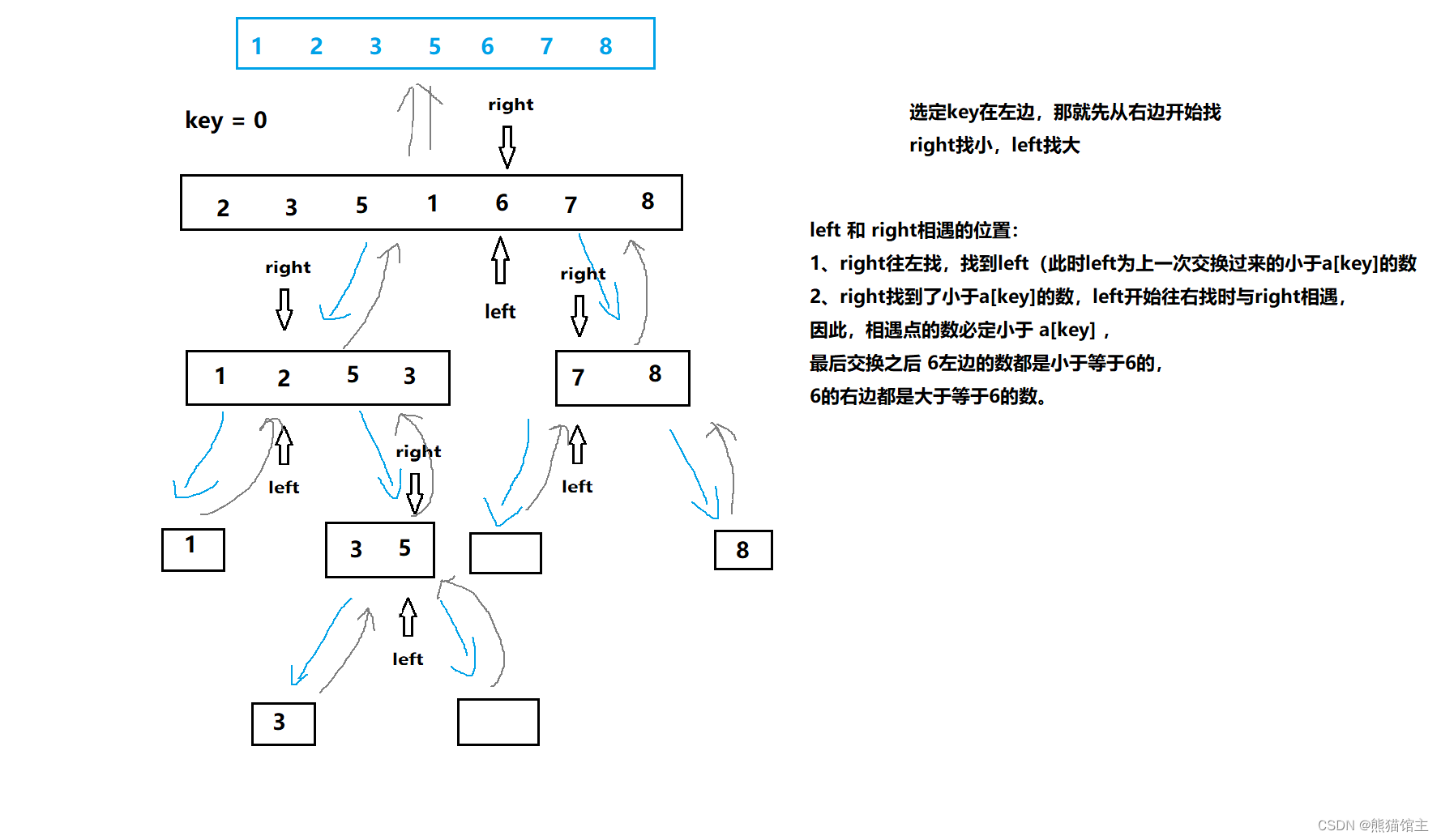``````// 快速排序hoare版本      left 与 right 都为下标
int PartSort1(int* a, int left, int right)
{int keyi = left;    //  key值while (left < right){if (a[right] >= a[keyi])right--;elseif (a[left] <= a[keyi])left++;if (a[right] < a[keyi] && a[left] > a[keyi])Swap(a + left, a + right);}Swap(a + right, a + keyi);   //  将key放到正确的位置keyi = right;return keyi;
}void QuickSort(int* a, int begin, int end)
{if (end <= begin)   //  下标需要合理return;int keyi = PartSort1(a, begin, end);QuickSort(a, begin, keyi - 1);  //  keyi - 1 有可能 小于 begin，下一个亦然，因此上面需要进行判断QuickSort(a, keyi + 1, end);}
``````

1. 通过类似二分的方式进行递归，每次将一个数据放到它应该在的位置，左边是小于等于x的数据，右边是大于等于x的数据。
2. 时间复杂度：O(n * logn)
3. 空间复杂度：O(logn) – 递归深度决定
4. 稳定性：不稳定

### 快速排序2（挖坑版）

1. 选取序列第一个位置为坑位，保留坑位数据作为目标值，保留坑位下标；
2. 从右边开始查找，选择小于key值的数据“填坑”，并将原位置作为新的坑位；
3. 从左边开始查找，选择大于key值的数据“填坑”，并将原位置作为新的坑位；
4. 重复2,3步操作直至两指针相遇，相遇点肯定是坑位，将key填入坑位；
5. 此时key左边的都是不大于key值的，右边都是不小于key值的，对左右两边分别重复上述操作。``````// 快速排序挖坑法
int PartSort2(int* a, int left , int right )
{int key = a[left ];  //  保留数据，留出坑位int keyi = left ;    //  坑位while (left < right){while (left < right && a[right] >= key)right--;a[keyi] = a[right];  // 执行到这一步的原因：keyi = right;  //  1.找到a[right]<key      2.left == right ，此时相遇在坑位，填不填坑没有影响，因此此处不需要再判断 left < right  while (left < right && a[left] < key)  //  不加等号：将相等的数据全部移动到侧left++;a[keyi] = a[left];keyi = left;}a[keyi] = key;return keyi;
}void QuickSort(int* a, int begin, int end)
{if (end <= begin)   //  下标需要合理return;int keyi = PartSort2(a, begin, end);QuickSort(a, begin, keyi - 1);  //  keyi - 1 有可能 小于 begin，下一个亦然，因此上面需要进行判断QuickSort(a, keyi + 1, end);}
``````

1. 通过类似二分的方式进行递归，每次将一个数据放到它应该在的位置，左边是小于等于x的数据，右边是大于等于x的数据。
2. 时间复杂度：O(n * logn)
3. 空间复杂度：O(logn) – 递归深度决定
4. 稳定性：不稳定

### 快速排序3（前后指针版）

1. 初始化两个指针，一个在起始位置，一个比它前一个位置；
2. 如果fast所指数据大于key（keyi所指数据），slow指针不动，slow指针以及它之前的数据都是不大于key的；
3. 前面的指针所指数据如果不大于key，并且不等于++slow（说明slow现在所指数据是大于key的）就交换；
4. 遍历直到fast大于right；``````// 快速排序前后指针法
int PartSort3(int* a, int left, int right)
{int keyi = left;     //  对比值int slow = left;int fast = left+ 1;while (++fast <= right)    //  取值区间[begin, end]{if (a[fast] <= a[keyi] && ++slow != fast)Swap(a + fast, a + slow);}Swap(a + keyi, a + slow);  //  slow以及它左边的都是小于key值的，右边的都是大于key值的keyi = slow;return keyi;
}void QuickSort(int* a, int begin, int end)
{if (end <= begin)   //  下标需要合理return;int keyi = PartSort3(a, begin, end);QuickSort(a, begin, keyi - 1);  //  keyi - 1 有可能 小于 begin，下一个亦然，因此上面需要进行判断QuickSort(a, keyi + 1, end);}
``````

1. 通过类似二分的方式进行递归，每次将一个数据放到它应该在的位置，左边是小于等于x的数据，右边是大于等于x的数据。
2. 时间复杂度：O(n * logn)
3. 空间复杂度：O(logn) – 递归深度决定
4. 稳定性：不稳定

### 优化

#### ①三数取中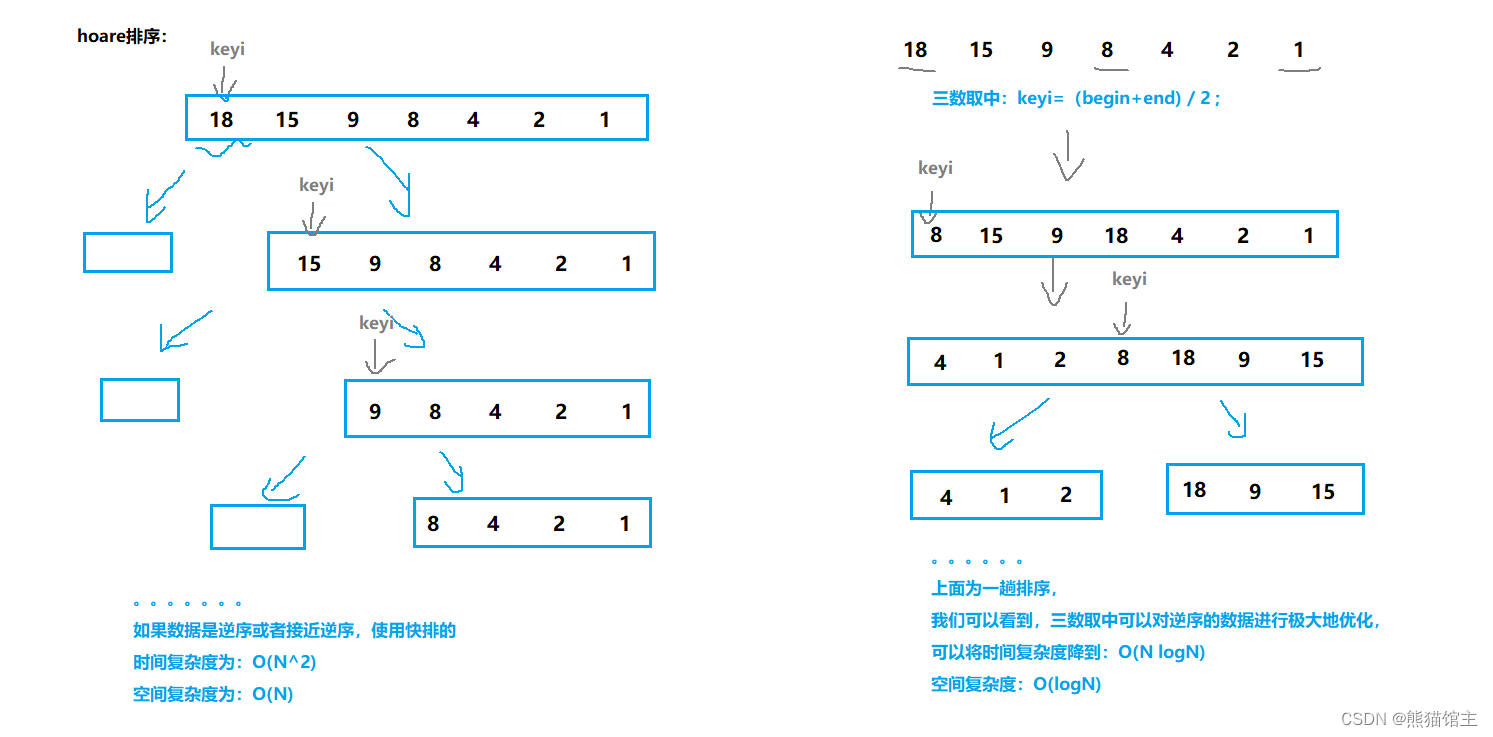``````//  优化1.1 -- 三数取中
int GetMidIndex(int* a, int begin, int end)
{int mid = (begin + end) >> 1;if (a[begin] > a[mid]){if (a[mid] >= a[end])return mid;else  //  a[mid] < a[end]{if (a[begin] > a[end])return end;elsereturn begin;}}else  //  a[begin] <= a[mid]{if (a[mid] <= a[end])return mid;else  //  a[mid] > a[end]{if (a[begin] > a[end])return begin;elsereturn end;}}
}
``````

#### ②小区间优化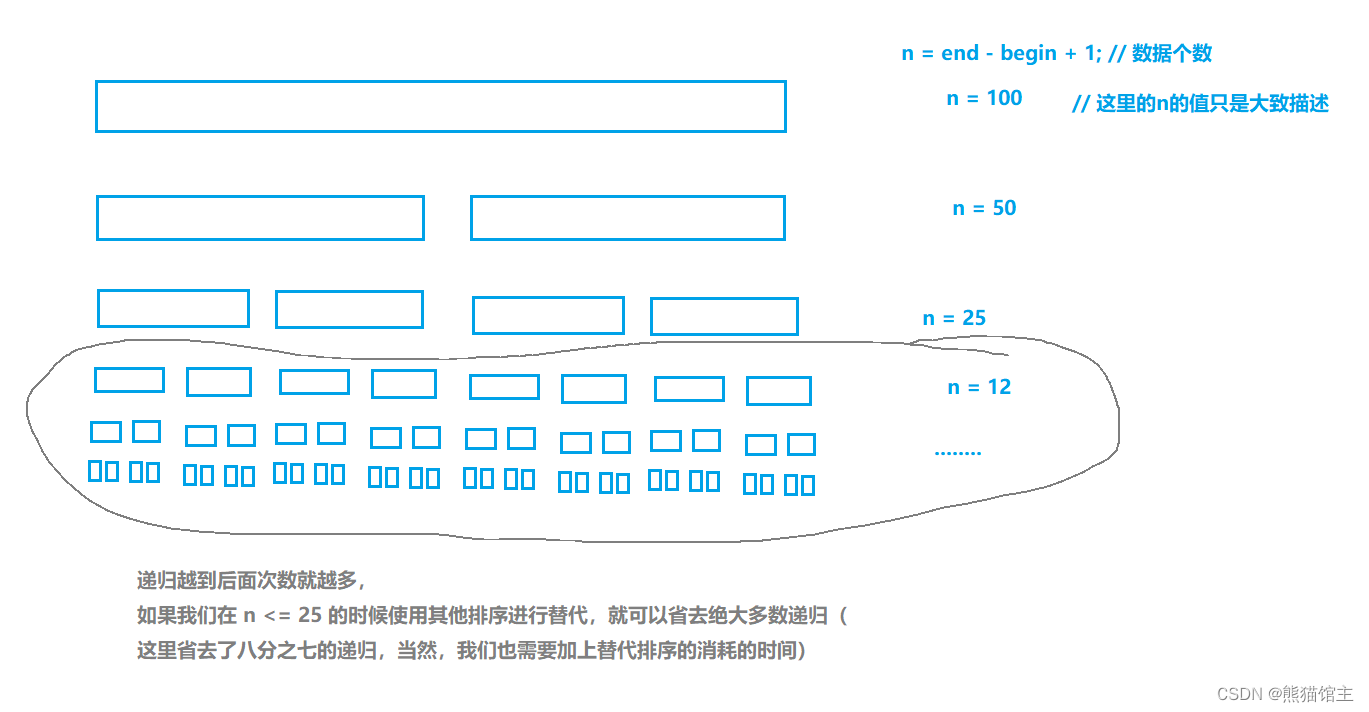``````if (st._end - st._begin + 1 <= 25)   //   熊猫选择插入排序InsertSort(a + st._begin, st._end - st._begin + 1);else{。。。。。。。}
``````

### 快速排序4（非递归版）

test.h

``````// 支持动态增长的栈
typedef struct interval
{int _begin;     //  起始和结束位置下标int _end;
}STDataType;
typedef struct Stack
{STDataType* _a;int _top;		// 栈顶int _capacity;  // 容量
}Stack;// 初始化栈
void StackInit(Stack* ps);
// 入栈
void StackPush(Stack* ps, STDataType data);
// 出栈
void StackPop(Stack* ps);
// 获取栈顶元素
STDataType StackTop(Stack* ps);
// 检测栈是否为空，如果为空返回非零结果，如果不为空返回0
bool StackEmpty(Stack* ps);
// 销毁栈
void StackDestroy(Stack* ps);
``````

test.c

``````// 初始化栈
void StackInit(Stack* ps)
{assert(ps);ps->_a = (STDataType*)malloc(sizeof(STDataType) * 3);ps->_top = 0;ps->_capacity = 3;
}
// 入栈
void StackPush(Stack* ps, STDataType data)
{assert(ps);if (ps->_top == ps->_capacity){STDataType* tmp = realloc(ps->_a, sizeof(STDataType) * ps->_capacity * 2);assert(tmp);ps->_a = tmp;ps->_capacity *= 2;}ps->_a[ps->_top++] = data;
}
// 出栈
void StackPop(Stack* ps)
{assert(ps);if (StackEmpty(ps))exit(-1);ps->_top--;
}
// 获取栈顶元素
STDataType StackTop(Stack* ps)
{assert(ps);if (StackEmpty(ps))exit(-1);return ps->_a[ps->_top - 1];
}
// 检测栈是否为空，如果为空返回非零结果，如果不为空返回0
bool StackEmpty(Stack* ps)
{assert(ps);return ps->_top == 0;
}
// 销毁栈
void StackDestroy(Stack* ps)
{assert(ps);free(ps->_a);ps->_a = NULL;ps->_top = ps->_capacity = 0;
}// 快速排序 非递归实现
void QuickSortNonR(int* a, int begin, int end)
{Stack S;StackInit(&S);STDataType st;st._begin = begin;st._end = end;StackPush(&S, st);while (!StackEmpty(&S)){st = StackTop(&S);  //  每次取栈顶数据，排序该区间StackPop(&S);if (st._end - st._begin + 1 < 15)InsertSort(a + st._begin, st._end - st._begin + 1);else{int mid = GetMidIndex(a, st._begin, st._end);Swap(a + st._begin, a + mid);int keyi = PartSort3(a, st._begin, st._end);if (keyi - 1 > begin){st._begin = keyi + 1;    // 右区间入栈st._end = st._end;StackPush(&S, st);}if (end > keyi + 1){st._begin = st._begin;   //  左区间入栈st._end = keyi - 1;StackPush(&S, st);}}}StackDestroy(&S);
}
``````

1. 非递归实现避免了数据量过大而导致的栈溢出。
2. 时间复杂度：O(N logN)
3. 空间复杂度：O(1)
4. 稳定性：不稳定

## （四）归并排序

### 递归版

1. 通过不断递归将数组分为一个个单独的数据；
2. 逆向合并数据，单个数据可以看做有序，合并两个有序数组；
3. 通过递归的返回，最终可以将所有数据合并为一个有序数组。
4. 注意：在合并的时候如果在原数组进行合并可能会覆盖数据，因此需要有一个临时数组存放合并的数据，合并结束后再拷贝回原数组。``````// 归并排序递归实现    时间复杂度：O(N*logN)    空间复杂度：O(N)  --   分区间操控，
void _MergeSort(int* a, int begin, int end, int* tmp)
{if (end <= begin)return;int mid = (begin + end) / 2;  //  将该区间分为两部分继续递归拆分_MergeSort(a, begin, mid, tmp);_MergeSort(a, mid + 1, end, tmp);int left1 = begin, right1 = mid;int left2 = mid + 1, right2 = end; int t = 0;  //  tmp数组下标while (left1 <= right1 && left2 <= right2)  //  合并两个有序数组{if (a[left1] <= a[left2])tmp[t++] = a[left1++];elsetmp[t++] = a[left2++];}while (left1 <= right1)tmp[t++] = a[left1++];while (left2 <= right2)tmp[t++] = a[left2++];  memcpy(a + begin, tmp, sizeof(tmp) * t);  // 将排好序的数据拷贝回原数组
}void MergeSort(int* a, int n)
{int* tmp = (int*)malloc(sizeof(int) * n);_MergeSort(a, 0, n - 1, tmp);free(tmp);
}
``````

1. 归并排序通过不断二分，时间复杂度为O(NlogN)，而且归并排序不受数据的影响，每次都会递归到最后之后合并，不过需要一个辅助数组来存放数据，因此空间复杂度为O(N)。
2. 时间复杂度：O(N logN)
3. 空间复杂度：O(N)
4. 稳定性：稳定

### 非递归版

``````// 归并排序非递归实现   --  手动设置排序区间，可以认为是希尔排序的相反思路 -- 熊猫是这么理解的
void MergeSortNonR(int* a, int n)
{int* tmp = (int*)malloc(sizeof(int) * n);int range = 1; // 排序区间中元素个数while (range < n){for (int i = 0; i < n; i += 2 * range){int left1 = i, right1 = left1 + range - 1;int left2 = i + range, right2 = left2 + range - 1;int t = 0;  //  tmp数组下标//  right1 left2 right2 越界if (right1 >= n)  break;elseif (left2 >= n)break;elseif (right2 >= n)right2 = n - 1;while (left1 <= right1 && left2 <= right2)  //  合并两个有序数组{if (a[left1] <= a[left2])tmp[t++] = a[left1++];elsetmp[t++] = a[left2++];}while (left1 <= right1)tmp[t++] = a[left1++];while (left2 <= right2)tmp[t++] = a[left2++];memcpy(a + i, tmp, sizeof(tmp) * t);  // 将排好序的数据拷贝回原数组  -- 每次排好序都进行拷贝}range *= 2;}free(tmp);
}
``````

2. 时间复杂度：O(N logN)
3. 空间复杂度：O(N)
4. 稳定性：稳定

## （五）计数排序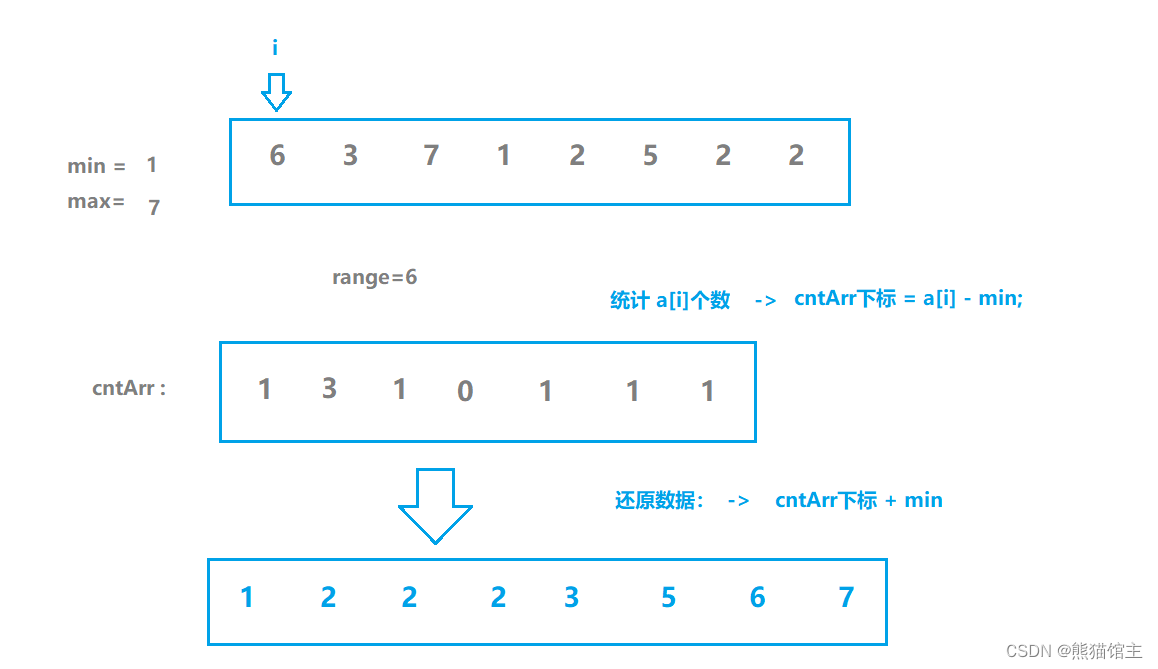``````// 计数排序
void CountSort(int* a, int n)
{int max = a;int min = a;for (int i = 1; i < n; ++i){if (a[i] > max)max = a[i];if (a[i] < min)min = a[i];}int range = max - min + 1; // 空间区间int* cntArr = (int*)calloc(range , sizeof(int));// 最小数  -- d - min      最大数   --  d  - minfor (int i = 0; i < n; ++i)  // 统计个数{++cntArr[a[i] - min];}int size = 0; // a数组下标for (int i = 0; i < range ; ++i)  // 导出排序数组{while (cntArr[i]--){a[size++] = min + i;}}free(cntArr);
}
``````

1. 计数排序适合数据集中的数据，并且，计数排序只适用于整形。
2. 时间复杂度：O(N+range)
3. 空间复杂度：O(range)
4. 稳定性：不稳定

# 三、性能分析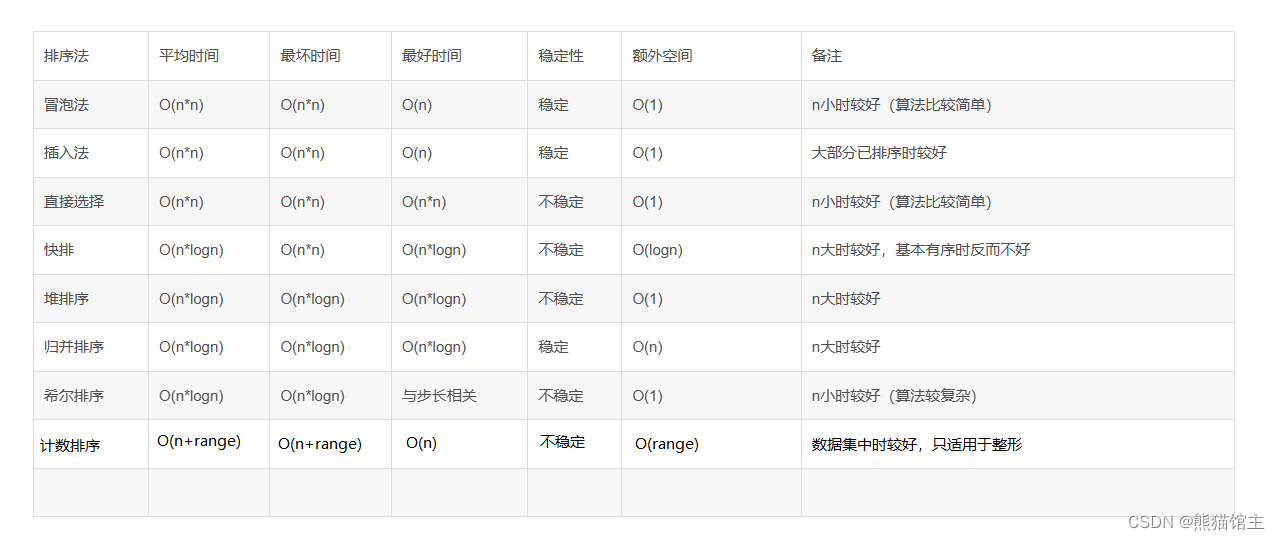# 总结

### 常用分析模型---AARRR分析法

AARRR模型 获取用户&#xff08;Acquisition&#xff09; 通过不同的渠道让用户下载APP&#xff0c;获取用户 激活用户&#xff08;Activation&#xff09; 通过提醒通知等功能&#xff0c;激活用户 提高留存&#xff08;Retention&#xff09; 培养用户习惯与粘性&#xff0c…